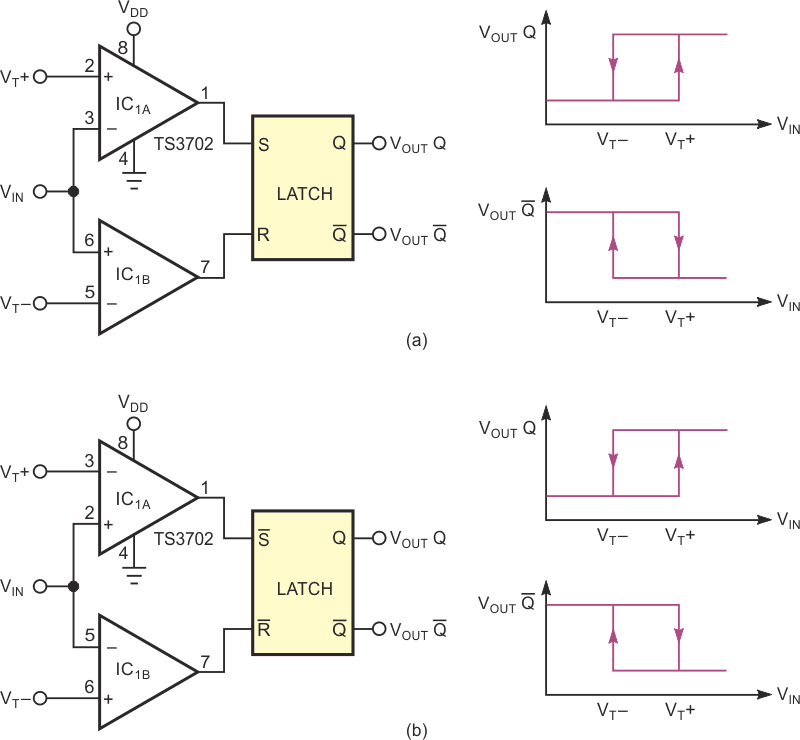# Add hysteresis to a voltage comparator

## STMicroelectronics TS3702

Positive feedback is a typical technique for distributing hysteresis around a comparator, provided that you have a resistive path between the comparator's output and the noninverting input. Positive feedback forms two threshold voltages that have (or assume) fixed values. In addition, they depend on the saturation values of the comparator's output stage. Plus, the load conditions affect their accuracy. The circuit in Figure 1 provides an alternative for applications requiring a comparator with hysteresis that has precise thresholds that you can easily and independently set. The circuit includes two inverting and noninverting threshold comparators whose outputs directly drive a set/reset latch. You can use a latch with either active-low or active-high inputs.Figure 1. The set/reset latch provides hysteresis for the comparator. The circuit provides a noninverting characteristic on the Q output and an inverting characteristic on the /Q output (a and b). Exchange the comparator outputs with the latch inputs for both circuits: The upper comparator drives the reset input, and the lower comparator drives the set input, and you get an inverting characteristic on the Q output and a noninverting characteristic on the /Q output.

You can generate the positive and negative threshold voltages using a precision voltage reference that powers a resistor divider (not shown) or by driving the inputs with DACs if you need a digitally programmable comparator. The circuit's high input impedance facilitates this task. Because of its hold state, the latch nullifies the effects of frequent switching on the comparator's outputs due to noise on the input signal. The circuit thus acts as a Schmitt trigger even if there is no positive feedback. The latch introduces a propagation delay that's normally a few tens of nanoseconds and is negligible in low- to medium-speed applications. Because the latch has complementary outputs, the circuit provides a noninverting characteristic on the Q output and an inverting characteristic on the /Q output (Figure 1a and Figure 1b).

Some integrated latches have only the Q output. If you need an inverted output, you need only to exchange the comparator outputs with the latch inputs for both circuits; the upper comparator drives the reset input, and the lower comparator drives the set input. You can use open-collector or open-drain comparators to process bipolar or positive signals higher than the supply voltage of the latch. You can easily interface them without using clamping diodes. You must add only a pullup resistor that the logic supply powers.

The circuit uses IC1, a dual micropower comparator TS3702 with a push-pull output stage. In this case, the supply voltage must be the same as that for the latch.

EDN